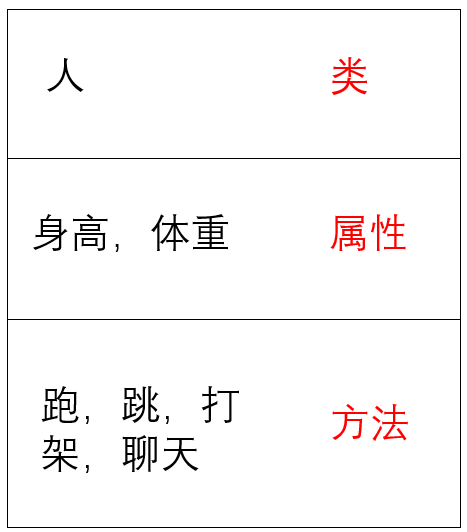设为首页 加入收藏
TOP
Python语法基础-函数，类以及调试处理(一)
2019-01-11 16:08:46 】 浏览:136
 Tags：Python 语法 基础 函数 以及 调试 处理

[TOC]

# 1. 函数的定义

python中函数有两种：

• python自带的函数
• 用户定义函数

## 1.1函数的参数

• 顺序： 必选参数<---默认参数<---可变参数<---命名关键字参数<---关键字参数
``````# 关键字参数
def person(name, age, **kw):
print('name:', name, 'age:', age, 'other:', kw)

person('hao', 20) # name: Michael age: 30 other: {}
person('hao', 20, gener = 'M', job = 'Engineer') # name: Adam age: 45 other: {'gender': 'M', 'job': 'Engineer'}
extra = {'city': 'Beijing', 'job': 'Engineer'}
person('Jack', 24, **extra)

# 命名关键字参数
def person(name, age, *, city='Beijing', job):
print(name, age, city, job)

person('Jack', 24, job = '123')
person('Jack', 24, city = 'Beijing', job = 'Engineer')

# Combination
# 可变 + 关键字参数
def f1(a, b, c=0, *args, **kw):
print('a =', a, 'b =', b, 'c =', c, 'args =', args, 'kw =', kw)

f1(1, 2, 3, 'a', 'b')   # a = 1 b = 2 c = 3 args = ('a', 'b') kw = {'x': 99}
f1(1, 2, 3, 'a', 'b', x=99) # a = 1 b = 2 c = 0 d = 99 kw = {'ext': None}

# 默认参数 + 命名关键字参数 + 关键字参数
def f2(a, b, c=0, *, d, **kw):
print('a =', a, 'b =', b, 'c =', c, 'd =', d, 'kw =', kw)

f2(1, 2, d=99, ext=None) # a = 1 b = 2 c = 0 d = 99 kw = {'ext': None}
``````

# 2. 面向对象编程

• 面向过程: 根据业务逻辑从上到下写代码
• 面向对象： 对数据与函数绑定到一起，进行封装，这样更快速的开发过程，减少代码重复使用

## 2.1. 类(抽象概念)和对象(具体概念)

• 类名：狗
• 类的属性：一组数据（狗的颜色，性别...）
• 类的方法： 运行进行操作的方法行为（行为，会叫，会咬人...）-> 用函数设计• 类是创建实例的模板，而实例则是一个一个具体的对象，各个实例拥有的数据都互相独立，互不影响；
• 方法就是与实例绑定的函数，和普通函数不同，方法可以直接访问实例的数据；
• 通过在实例上调用方法，我们就直接操作了对象内部的数据，但无需知道方法内部的实现细节。
• 继承可以把父类的所有功能都直接拿过来，这样就不必重零做起，子类只需要新增自己特有的方法，也可以把父类不适合的方法覆盖重写。

### (1). 定义类

``````# 定义类
class Dog(object)：

# 定义初始化方法
def __init__(self,weight,color):
"""
self: python会把对象的地址自动传给self，不要自己传
weight, color： 接收外部属性
"""
# 定义属性
self.weight = weight
self.color = color

# 魔法方法： 当只打印Dog对象的时候，就可以打印这里的东西
def __str__(self):
msg = "dog weight" + self.weight + "color" + self.color
return "哈哈哈"

def getweight(self):
return self.weight
def getcolor(self):
return self.color
def setweight(self):
self.weight = 100
def setcolor(self):
self.color = "green"

# 定义方法
def bark(self):
"""
self: python解释器默认把调用该class的对象地址给它
"""
print("666")

def run(self):
print("777")

# 创建对象
huskey = Dog(5, 'Black')  # 创建一个哈士奇
keji = Dog(10, 'Green')

huskey.bark()  # 哈士奇叫
huskey.run()   # 哈士奇跑
huskey.weight = 100  # 哈士奇属性
huskey.color = 'green'

``````
• `self`表示自己，表示调用类的对象本身
• python中类似 `__***__`的方法，是魔法方法，有特殊用途

### (2). 类的数据封装

``````class Dog(object):

def __init__(self, weight, color):
self.weight = weight
self.color = color

def print_dog(self):
print('%s: %s' % (self.weight, self.color))
``````

### (3). 访问限制

】【打印繁体】【投稿】【收藏】 【推荐】【举报】【评论】 【关闭】 【返回顶部array(4) { ["type"]=> int(8) ["message"]=> string(24) "Undefined variable: jobs" ["file"]=> string(32) "/mnt/wp/cppentry/do/bencandy.php" ["line"]=> int(217) }